# Semi-supervised learning and its goal

Last updated on：a year ago

Semi-supervised learning is a learning paradigm concerned with how to learn the presence of both labelled and unlabelled data.

# Introduction

Semi-supervised learning is an approach to machine learning that combines a small amount of labelled data with many unlabelled data during training. It falls between unsupervised learning and supervised learning.

## Motivation

• Unlabelled data is easy to be obtained.

• Labelled data can be hard to get.

• Labelled data sometimes is scarce or expensive.

Semi-supervised learning is of great interest in machine learning and data mining because it can use readily available unlabelled data to improve supervised learning tasks.

## Goal

Semi-supervised learning mixes labelled and unlabelled data to produce better models.

Semi-supervised learning aims to understand how combining labelled, and unlabelled data may change the learning behaviour, and design algorithms that take advantage of such a combination.

Two distinct goals: (a) predict the labels on future test data **(inductive semi-supervised learning). (b) predict the labels on the unlabelled instances in the training example **(transductive learning).

## The data point of view

Data in both input $x$ and output $y$ with known partial mapping (learn the mapping $f$)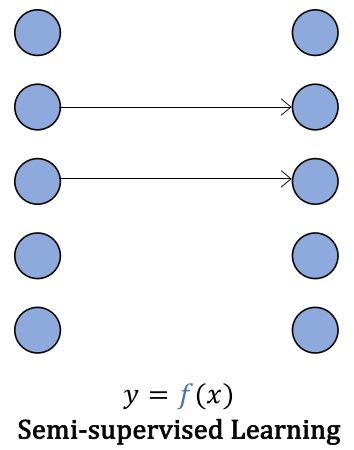PNU learning. P: positive data, N: negative data, U: unlabelled data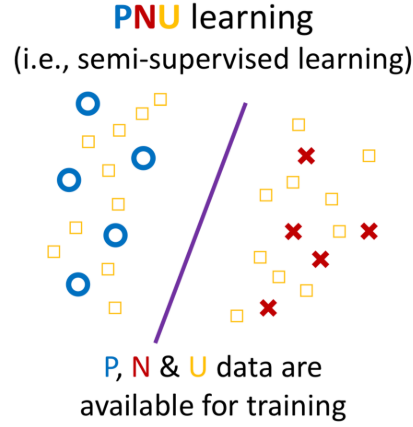## How does semi-supervised learning work?

Instances in each class form a coherent group (e.g., $p(\bf{x}|y)$) is a Gaussian distribution, such that the instances from each class centre around a central mean. The distribution of unlabelled data helps identify regions with the same label, and the few labelled data then provide the actual labels.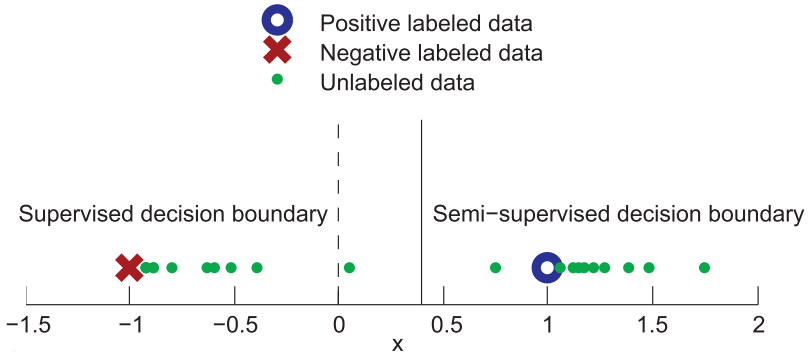Unlabelled data can help to find a better boundary.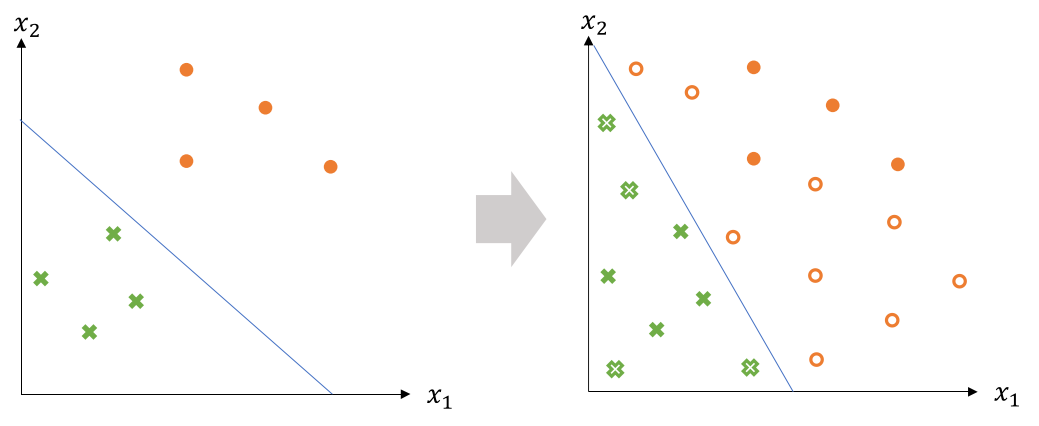# Semi-supervised Learning Methods

Semi-supervised learning is of great interest in machine learning and data mining because it can use readily available unlabelled data to improve supervised learning tasks.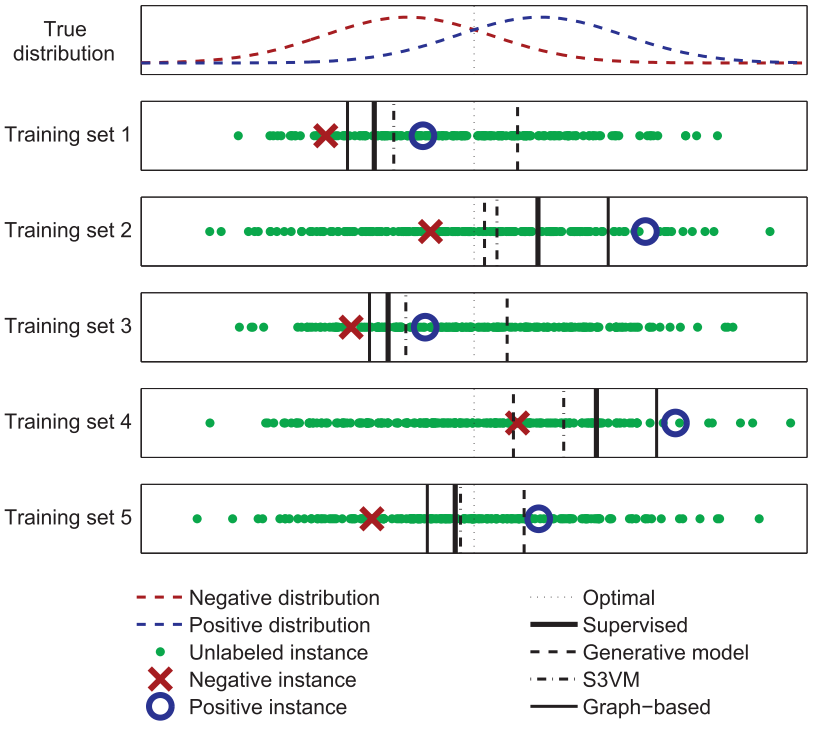## Pseudo Labelling

Pseudo means not genuine, spurious or sham.

Pseudo labelling is adding confidently predicted test data to our training data. Pseudo labelling is a 5 step process:

(1) Build a model using training data.

(2) Predict labels for an unseen test dataset.

(3) Add confidently predicted test observations to our training data.

(4) Build a new model using combined data.

(5) Use your new model to predict the test data.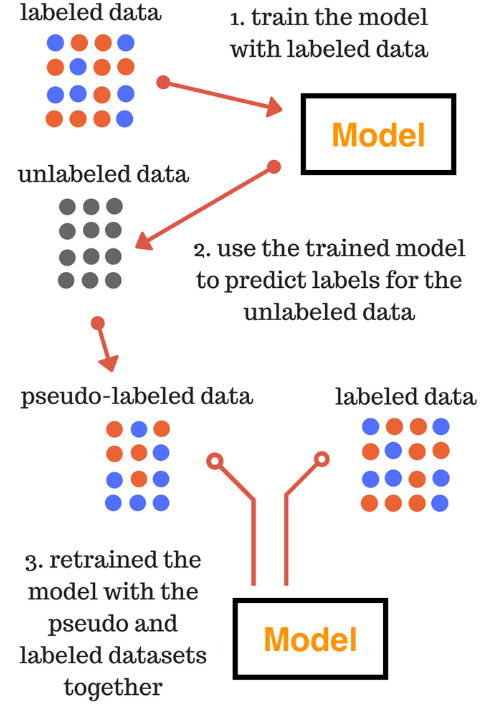### Self-training

Pseudo labelling is widely used in self-training. Self-training is one of the most used semi-supervised methods.

Self-training algorithms is to learn a classifier iteratively by assigning pseudo-labels to the set of unlabelled training samples with a margin greater than a certain threshold. The pseudo-labelled examples are then used to enrich the labelled training data and train a new classifier in conjunction with the labelled training set.

Liu et al. used one point on a subject to generate the initial pseudo labels. They use a super-voxel graph to propagate labels over the point cloud to further iteratively update the predicted labels.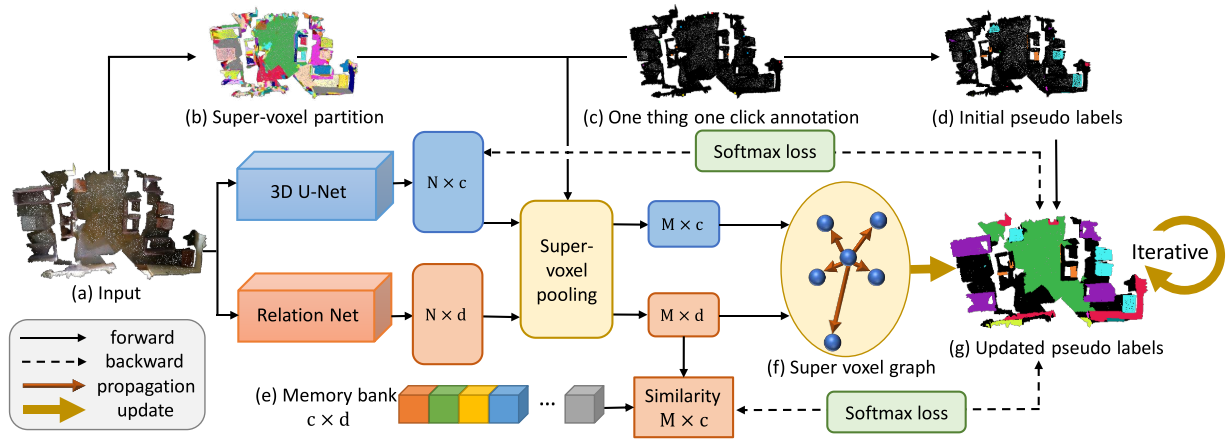### Application

Classification

Task-specific self-training: the model predicts class probabilities for the unlabelled samples as the pseudo-label to train again.

Task-agnostic self-supervised training: the model projects samples into low-dimensional embeddings and performs contrastive learning to discriminate embeddings of different images.

CoMatch: class probabilities and embeddings interact and jointly evolve in a co-training framework. The embedding imposes a smoothness constraint on the class probabilities to improve the pseudo-labels.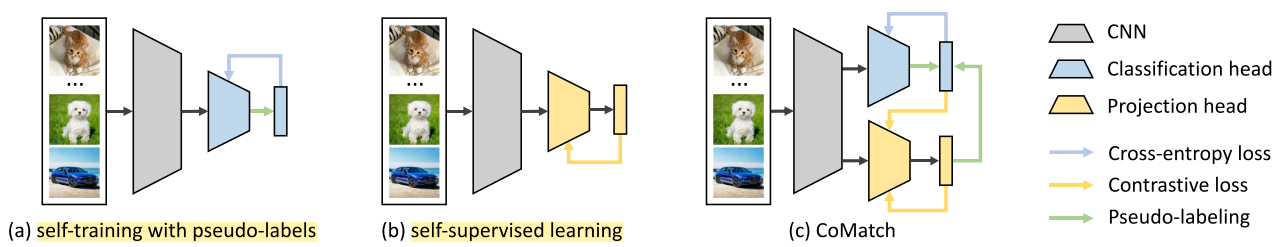Codes:

Training:

ims_x_weak, lbs_x = next(dl_x)
(ims_u_weak, ims_u_strong0, ims_u_strong1), lbs_u_real = next(dl_u)
lbs_x = lbs_x.cuda()
lbs_u_real = lbs_u_real.cuda()
# --------------------------------------
bt = ims_x_weak.size(0)
btu = ims_u_weak.size(0)
imgs = torch.cat([ims_x_weak, ims_u_weak, ims_u_strong0, ims_u_strong1], dim=0).cuda()
logits, features = model(imgs)
logits_x = logits[:bt]
logits_u_w, logits_u_s0, logits_u_s1 = torch.split(logits[bt:], btu)
feats_x = features[:bt]
feats_u_w, feats_u_s0, feats_u_s1 = torch.split(features[bt:], btu)
loss_x = criteria_x(logits_x, lbs_x)

logits_u_w = logits_u_w.detach()
feats_x = feats_x.detach()
feats_u_w = feats_u_w.detach()

probs = torch.softmax(logits_u_w, dim=1)
# DA
prob_list.append(probs.mean(0))
if len(prob_list)>32:
prob_list.pop(0)
prob_avg = torch.stack(prob_list,dim=0).mean(0)
probs = probs / prob_avg
probs = probs / probs.sum(dim=1, keepdim=True)
probs_orig = probs.clone()

if epoch>0 or it>args.queue_batch: # memory-smoothing
A = torch.exp(torch.mm(feats_u_w, queue_feats.t())/args.temperature)
A = A/A.sum(1,keepdim=True)
probs = args.alpha*probs + (1-args.alpha)*torch.mm(A, queue_probs)

scores, lbs_u_guess = torch.max(probs, dim=1)

feats_w = torch.cat([feats_u_w,feats_x],dim=0)
onehot = torch.zeros(bt,args.n_classes).cuda().scatter(1,lbs_x.view(-1,1),1)
probs_w = torch.cat([probs_orig,onehot],dim=0)

# update memory bank
n = bt+btu
queue_feats[queue_ptr:queue_ptr + n,:] = feats_w
queue_probs[queue_ptr:queue_ptr + n,:] = probs_w
queue_ptr = (queue_ptr+n)%args.queue_size

Embedding similarity;

sim = torch.exp(torch.mm(feats_u_s0, feats_u_s1.t())/args.temperature)
sim_probs = sim / sim.sum(1, keepdim=True)

Contrastive loss:

loss_contrast = - (torch.log(sim_probs + 1e-7) * Q).sum(1)
loss_contrast = loss_contrast.mean()

Unsupervised classification loss:

loss_u = - torch.sum((F.log_softmax(logits_u_s0,dim=1) * probs),dim=1) * mask
loss_u = loss_u.mean()
loss = loss_x + args.lam_u * loss_u + args.lam_c * loss_contrast

Pseudo-label graph with self-loop:

Q = torch.mm(probs, probs.t())
Q.fill_diagonal_(1)
Q = Q / Q.sum(1, keepdim=True)

pos_meter.update(pos_mask.sum(1).float().mean().item())

Segmentation

There are two different models trained on the labelled subset, and one model provides pseudo supervisions for the other.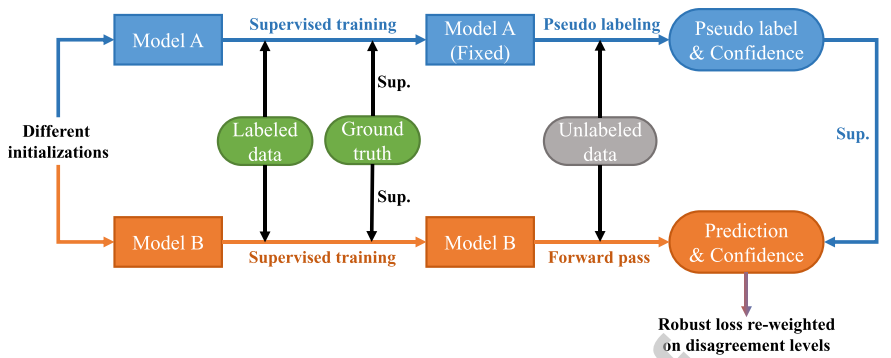Ke et al. generate a pseudo mask for unlabelled data while enforcing segmentation consistency in a multi-task fashion. (rough masks - > higher quality masks)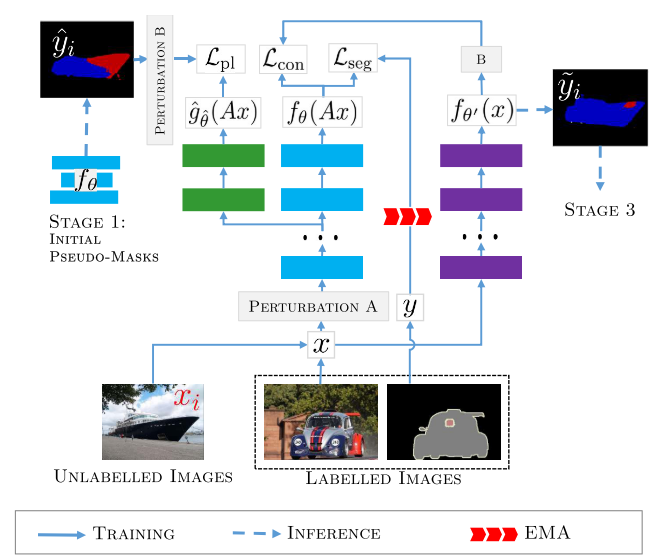Localization

The peripheral branch is first trained in the warm-up epoches. After that, the peripheral branch supervises main branch by its loss and output label.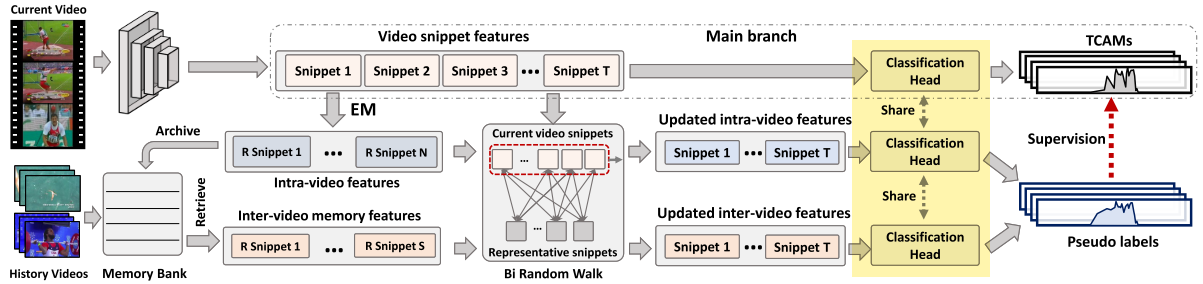Codes:

for num, sample in enumerate(self.train_data_loader):
if self.args.decay_type == 0:
for param_group in self.optimizer_module.param_groups:
param_group['lr'] = current_lr
elif self.args.decay_type == 1:
if num == 0:
current_lr = self.Step_decay_lr(epoch)
for param_group in self.optimizer_module.param_groups:
param_group['lr'] = current_lr
elif self.args.decay_type == 2:
current_lr = self.Cosine_decay_lr(epoch, num)
for param_group in self.optimizer_module.param_groups:
param_group['lr'] = current_lr
iter = iter + 1
np_features = sample['data'].numpy()
np_labels = sample['labels'].numpy()
labels = torch.from_numpy(np_labels).float().to(self.device)
features = torch.from_numpy(np_features).float().to(self.device)
f_labels = torch.cat([labels, torch.zeros(labels.size(0), 1).to(self.device)], -1)
b_labels = torch.cat([labels, torch.ones(labels.size(0), 1).to(self.device)], -1)
# the output and loss of peripheral branch will be used to supervise main branch
o_out, m_out, em_out = self.model(features)
vid_fore_loss = self.loss_nce(o_out, f_labels) + self.loss_nce(m_out, f_labels)
vid_back_loss = self.loss_nce(o_out, b_labels) + self.loss_nce(m_out, b_labels)
vid_att_loss = self.loss_att(o_out)
# use warm-up epoch only in peripheral branch
if epoch > self.args.warmup_epoch:
idxs = np.where(np_labels==1).tolist()
cls_mu = self.memory._return_queue(idxs).detach()
reallocated_x = random_walk(em_out, cls_mu, self.args.w)
r_vid_ca_pred, r_vid_cw_pred, r_frm_fore_att, r_frm_pred = self.model.PredictionModule(reallocated_x)
vid_fore_loss += 0.5 * self.loss_nce(r_vid_ca_pred, f_labels)
vid_back_loss += 0.5 * self.loss_nce(r_vid_cw_pred, b_labels)
vid_spl_loss = self.loss_spl(o_out, r_frm_pred * 0.2 + m_out * 0.8)
self.memory._update_queue(em_out.squeeze(0), em_out.squeeze(0), idxs)
else:
vid_spl_loss = self.loss_spl(o_out, m_out)
total_loss = vid_fore_loss + vid_back_loss * self.args.lambda_b \
+ vid_att_loss * self.args.lambda_a + vid_spl_loss * self.args.lambda_s
loss_recorder['cls_fore'] += vid_fore_loss.item()
loss_recorder['cls_back'] += vid_back_loss.item()
loss_recorder['att'] += vid_att_loss.item()
loss_recorder['spl'] += vid_spl_loss.item()
total_loss.backward()

Detection

A teacher model is employed during each training iteration to produce pseudo-labels for weakly augmented unlabelled images.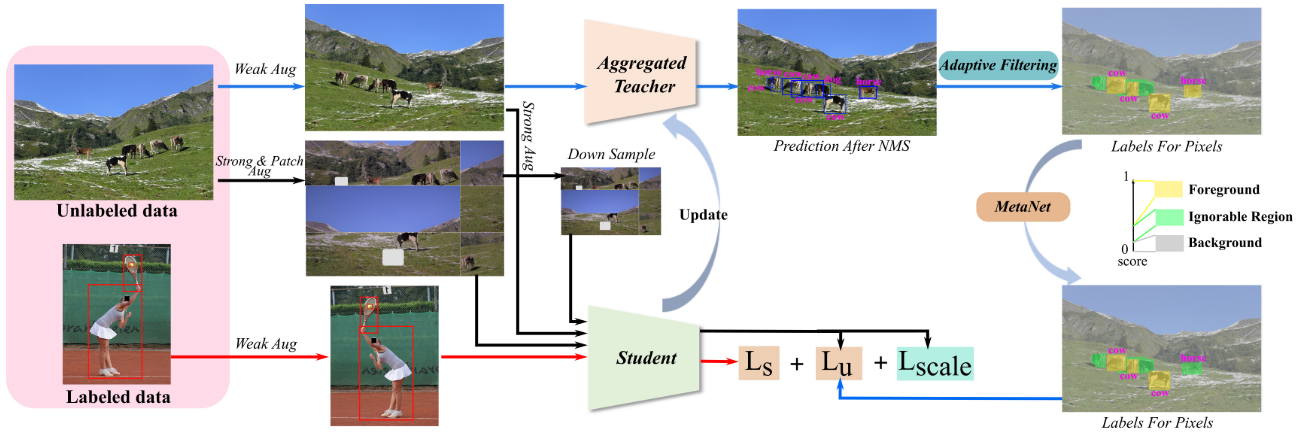## Generative methods (GE)

Unlabelled data shows how the instances from all the mixed together classes, are distributed.

EM with some labelled data: cluster and then label.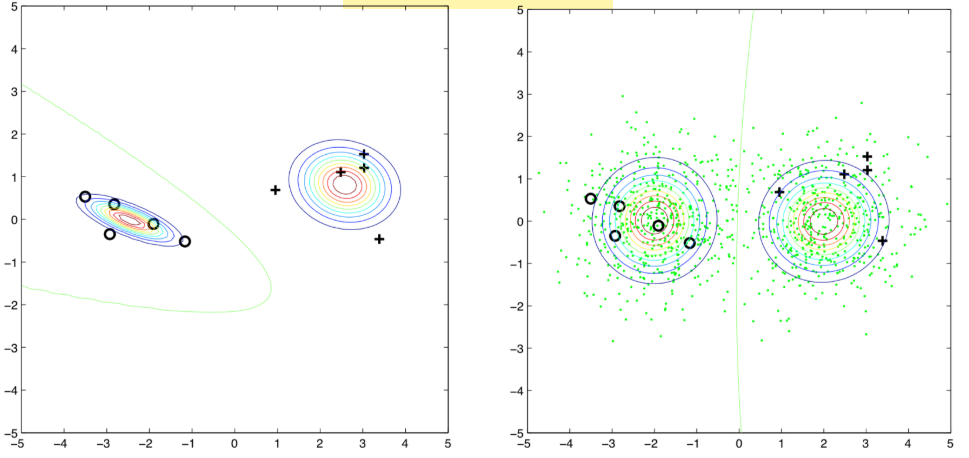Unlabelled data may hurt the learning.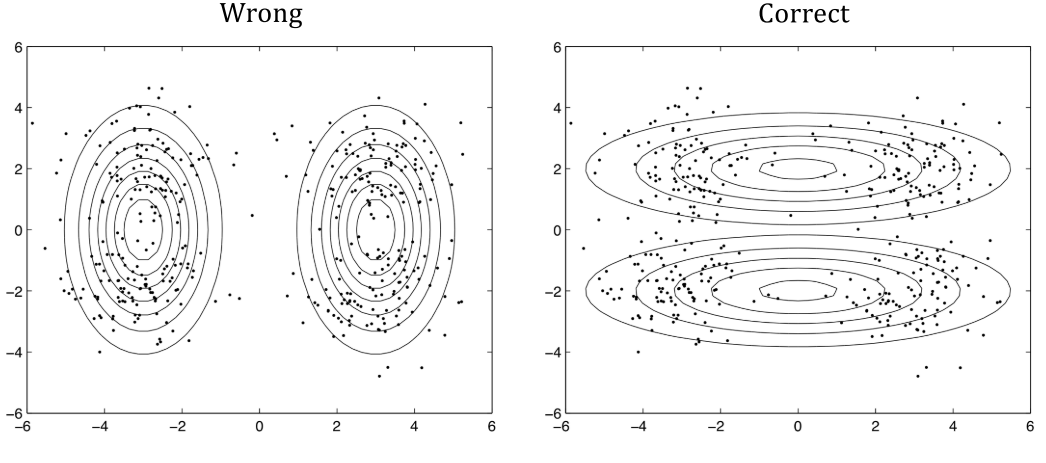## Graph-based methods

(1) Define the similarity $s(x_i, x_j)$

(3) Edge weight is proportional to $s(x_i, x_j)$

(4) Propagate through the graph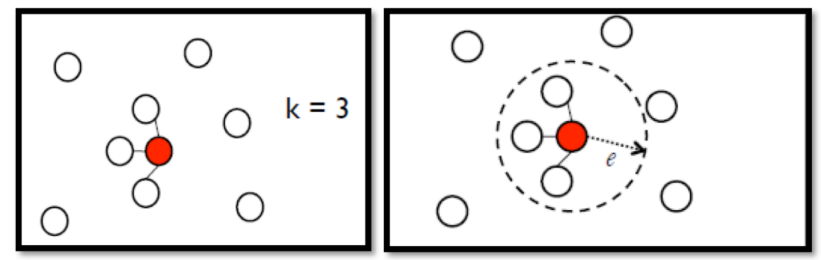# Reference

 Zhu, X. and Goldberg, A.B., 2009. Introduction to semi-supervised learning. Synthesis lectures on artificial intelligence and machine learning, 3(1), pp.1-130.

 Wiki, Semi-supervised learning.

 Hao Dong, Learning Methods.

 Kaggle, Pseudo Labelling.

 Chen, B., Li, P., Chen, X., Wang, B., Zhang, L. and Hua, X.S., 2022. Dense Learning based Semi-Supervised Object Detection. arXiv preprint arXiv:2204.07300.

 Li, J., Xiong, C. and Hoi, S.C., 2021. Comatch: Semi-supervised learning with contrastive graph regularization. In Proceedings of the IEEE/CVF International Conference on Computer Vision (pp. 9475-9484).

 Amini, M.R., Feofanov, V., Pauletto, L., Devijver, E. and Maximov, Y., 2022. Self-Training: A Survey. arXiv preprint arXiv:2202.12040.

 Huang, L., Wang, L. and Li, H., 2022. Weakly Supervised Temporal Action Localization via Representative Snippet Knowledge Propagation. arXiv preprint arXiv:2203.02925.

 LeonHLJ/RSKP